JEE  >  JEE Main Physics Mock - 7

# JEE Main Physics Mock - 7

Test Description

## 25 Questions MCQ Test JEE Main & Advanced Mock Test Series | JEE Main Physics Mock - 7

JEE Main Physics Mock - 7 for JEE 2023 is part of JEE Main & Advanced Mock Test Series preparation. The JEE Main Physics Mock - 7 questions and answers have been prepared according to the JEE exam syllabus.The JEE Main Physics Mock - 7 MCQs are made for JEE 2023 Exam. Find important definitions, questions, notes, meanings, examples, exercises, MCQs and online tests for JEE Main Physics Mock - 7 below.
Solutions of JEE Main Physics Mock - 7 questions in English are available as part of our JEE Main & Advanced Mock Test Series for JEE & JEE Main Physics Mock - 7 solutions in Hindi for JEE Main & Advanced Mock Test Series course. Download more important topics, notes, lectures and mock test series for JEE Exam by signing up for free. Attempt JEE Main Physics Mock - 7 | 25 questions in 60 minutes | Mock test for JEE preparation | Free important questions MCQ to study JEE Main & Advanced Mock Test Series for JEE Exam | Download free PDF with solutions
 1 Crore+ students have signed up on EduRev. Have you?
JEE Main Physics Mock - 7 - Question 1

### In a circuit, L, C and R are connected in series with an alternating voltage source of frequency f. The current leads the voltage by 45o. The value of C is

JEE Main Physics Mock - 7 - Question 2

### A hotwire ammeter reads 10 A in a a.c. circuit. The peak value of the current is

Detailed Solution for JEE Main Physics Mock - 7 - Question 2

Hot wire ammeter always gives rms value of current, i.e.,
Irms = 10 amp. as Irms = I0/√2

hence I= √2 Irms = √2 x 10 amp = 10√2 A

JEE Main Physics Mock - 7 - Question 3

### The average acceleration vector for a particle having a uniform circular motion is

Detailed Solution for JEE Main Physics Mock - 7 - Question 3 In uniform circular motion (UCM), the direction of the acceleration vector points toward the centre of the circle. However, it constantly changes with time. The average of these vectors over one cycle is a null vector.
JEE Main Physics Mock - 7 - Question 4
The X-rays beams coming from an X-ray tube will be
JEE Main Physics Mock - 7 - Question 5

A dipole of dipole moment p̅ is placed in uniform electric field E̅, then torque acting on it is given by

JEE Main Physics Mock - 7 - Question 6
When two surfaces are coated with a lubricant, then they
JEE Main Physics Mock - 7 - Question 7
Two pieces of a glass plate, placed one upon the other with a little water in between them, can not be separated easily, because of
JEE Main Physics Mock - 7 - Question 8

The gyromagnetic ratio of an electron of charge e and mass m is equal to

JEE Main Physics Mock - 7 - Question 9
If the momentum of a body increases by 0.01% its kinetic energy will increase by
JEE Main Physics Mock - 7 - Question 10

An electric dipole is placed along the X-axis at the origin O. A point P is at a distance of 20 cm from this origin such that OP makes an angle π/3 with the X axis. If electric field at P makes an angle θ with X-axis, the value of θ is

JEE Main Physics Mock - 7 - Question 11

In the following combinations of logic gates, the outputs of A, B and C are respectivley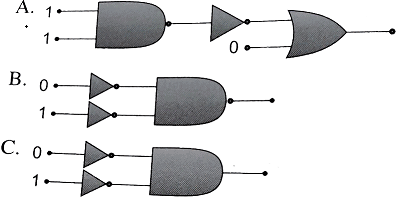JEE Main Physics Mock - 7 - Question 12
Find the maximum velocity for overturn for a car moved on a circular track of radius 100 m. The coefficient of friction between the road and tyre is 0.2
JEE Main Physics Mock - 7 - Question 13

An alpha nucleus of energy 1/2 mv2  bombards a heavy nuclear target of charge Ze. Then the distance of closest approach for the alpha nucleus will be proportional to

JEE Main Physics Mock - 7 - Question 14
In the following question, a Statement of Assertion (A) is given followed by a corresponding Reason (R) just below it. Read the Statements carefully and mark the correct answer-
Assertion(A): We cannot think of a magnetic configuration with three poles.
Reason(R): A bar magnet does exert a torque on itself due to its own field.
JEE Main Physics Mock - 7 - Question 15
In the following question, a Statement of Assertion (A) is given followed by a corresponding Reason (R) just below it. Read the Statements carefully and mark the correct answer-
Assertion(A): Gravitational field intensity at the centre of ring is zero.
Reason(R): Net force at the centre of ring is zero.
JEE Main Physics Mock - 7 - Question 16

A disc is of mass M and radius r. The moment of inertia of it about an axis tangential to its edge and in plane of the disc or parallel to its diameter is

JEE Main Physics Mock - 7 - Question 17

Helium gas at 27oC has a volume of 8 L. It is suddenly compressed to a volume of 1 L. The final temperature of the gas will be(γ = 5/3 )

Detailed Solution for JEE Main Physics Mock - 7 - Question 17

T1 V1 γ-1 = T2 V2 γ-1
or 300 (8)5/3 - 1 = T2 (1)5/3 - 1
T2 = 300 {(2)3}2/3 = 1200 K
or T2 = 927oC

JEE Main Physics Mock - 7 - Question 18
A tuning fork produces waves in a medium. If the temperature of the medium changes, then which of the following will change
JEE Main Physics Mock - 7 - Question 19
JEE Main Physics Mock - 7 - Question 20

The radionuclide 56Mn is being produced in a cyclotron at a constant rate P by bombarding a manganese target with deutrons. 56Mn has a half life of 2.5 hours and the target contains large number of only the stable manganese isotope 55Mn. The reaction that produces 56Mn is:

55Mn + d 56Mn+P

After being bombarded for a long time, the activity of 56Mn becomes constant equal to 13.86×1010s−1. (Use ln2 = 0.693; Avogadro number = 6×1023; atomic weight of 56Mn = 56gm/mole)

Q. After a long time bombardment, number of 56Mn nuclei present in the target depends upon
(a) The number of 56Mn nuclei present at the start of the process
(b) Half life of the 56Mn
(c) The constant rate of production P

*Answer can only contain numeric values
JEE Main Physics Mock - 7 - Question 21

After five half-lives percentage of original radioactive atoms left is:-

Detailed Solution for JEE Main Physics Mock - 7 - Question 21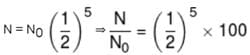*Answer can only contain numeric values
JEE Main Physics Mock - 7 - Question 22

In series LR circuit XL = 3R. Now a capacitor with XC = R is added in series. Ratio of new to old power factor is :-

Detailed Solution for JEE Main Physics Mock - 7 - Question 22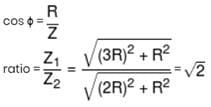*Answer can only contain numeric values
JEE Main Physics Mock - 7 - Question 23

In a galvanometer, the deflection becomes one half when the galvanometer is shunted by a 20Ω resistor. The galvanometer resistance is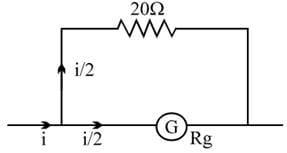Detailed Solution for JEE Main Physics Mock - 7 - Question 23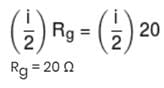*Answer can only contain numeric values
JEE Main Physics Mock - 7 - Question 24

If an objects is placed symmetrically between two plane mirrors, inclined at an angle of 72°, then the total number of images formed is :-

*Answer can only contain numeric values
JEE Main Physics Mock - 7 - Question 25

A tuning fork gives 4 beats with 50cm length of a sonometer wire. If the length of the wire is shortened by 1cm, the number of beats is still the same. The frequency of the fork (in Hz) is :-

Detailed Solution for JEE Main Physics Mock - 7 - Question 25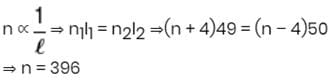## JEE Main & Advanced Mock Test Series

2 videos|324 docs|165 tests
 Use Code STAYHOME200 and get INR 200 additional OFF Use Coupon Code
Information about JEE Main Physics Mock - 7 Page
In this test you can find the Exam questions for JEE Main Physics Mock - 7 solved & explained in the simplest way possible. Besides giving Questions and answers for JEE Main Physics Mock - 7, EduRev gives you an ample number of Online tests for practice

## JEE Main & Advanced Mock Test Series

2 videos|324 docs|165 tests